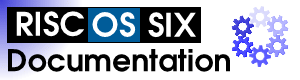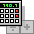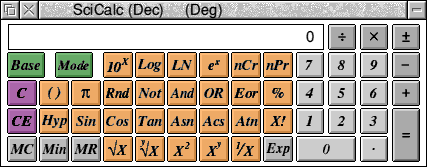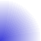## Application Suite: SciCalc#### SciCalcSciCalc is a scientific calculator which runs on the RISC OS desktop. To load SciCalc open the Apps folder on the iconbar and double click on the SciCalc icon. SciCalc will then appear on the RISC OS iconbar. Click on this icon with SELECT to open the calculator window.You can use SciCalc in two ways:

Firstly you can click on the buttons to enter numbers and perform calculations. In this mode SciCalc operates just like a real desktop calculator.

Secondly you can click in the display section of the SciCalc window (the box with 0 at the right hand end) with SELECT. This will give keyboard input focus to SciCalc. You will see that the title bar goes yellow. If the title bar is not yellow then SciCalc will not accept key presses from the keyboard. You can now type numbers on the numeric keypad on your keyboard and the numbers will be entered into SciCalc. You can also use the keys that have mathematical signs on them, for example the + (plus), - (minus), * (multiply) and / (divide) keys.

As an example suppose you wanted to add 2 numbers together. Type the first number of the numeric keypad, lets say 25. Then press the + (plus) key. Now type the second number, lets say 57.23 (use the full stop key to represent the decimal point). Now press the Enter key and SciCalc will show you the result of adding the two numbers.

The version of SciCalc supplied with RISC OS Six now supports cutting and pasting of values using the RISC OS clipboard from applications such as Edit. Use Ctrl-C to copy, and Ctrl-V to paste.

You will find that SciCalc is a very useful tool as you can always have a calculator available. SciCalc is also very useful for software developers and the following sections cover the more technical aspects.

SciCalc Technical Information

SciCalc offers 4 numeric bases, 3 trigonometric modes, a memory and a single level of brackets. Its accuracy is limited to that of BASIC 64, which SciCalc uses for calculations.

Numeric range

SciCalc has the following numeric ranges:

• Decimal Real values, -9.9E99 to 9.9E99 entry, (-9.9E307 to 9.9E307 displayable)
• Binary - 32bit signed, 10000000000000000000000000000000 to 11111111111111111111111111111111
• Octal - 32bit signed 20000000000 to 17777777777
• Hexadecimal - 32bit signed, 80000000 to 7FFFFFFF

Accuracy

```
Mode           Accuracy          Number of digits displayed

Binary         Absolute          32
Octal          Absolute          10 - 11
Decimal        64 bit FP         10 or 10 + 2 digit exponent
```

Note that real number underflow is not trapped.

Keys in decimal base

```  Calculator        Keyboard           Function

0..9              0..9               numbers
+, -, x, ¼, =,    +, -, *, /, =      standard operators
Return, Enter      same as =
Ý                 #                  change sign on number or exponent
Exp               E                  allows entry of exponent
.                 .                  decimal point
10x, LOG, LN, eX                     exponential and logarithmic functions
nCr                                  combination
nPr                                  permutation
()                (, )               brackets (one level only)
TT                                   the number Pi
RND                                  random number between 0.0 and 1.0
NOT, AND, OR, EOR                    logical functions
%                 %                  percentage
HYP (followed by trig fn)            hyperbolic functions
SIN, COS, TAN                        trigonometric functions
ASN, ACS, ATN                        inverse trigonometric functions
x!                !                  factorial
x                                   square root
yx                                  Yth root of X
xý                                   square
xY                ^                  X raised to the power Y
1/x                                  reciprocal
C                 Delete             clear calculation
CE                                   clear current number only
MC                                   clear memory
Min                                  memory in
MR                                   memory recall
Base                                 select decimal/binary/octal/
```

Alternative keys in non-decimal bases

```  NAND                          NOT AND
NOR                           NOT OR
EQV                           NOT EOR (equivalence)
<O , O>                 rotate left or right
<<< , >>>   logical shift left or right
<< , >>           arithmetic shift left or right
DIV                           integer division
MOD                           modulus (integer remainder)
A - F             A - F       hexadecimal base digits
```

Using the percentage key

Here are some examples of using the percentage key:

```47.3 + 15 % = take 47.3 and increase it by 15%
15 % * 47.3 = find 15% of 47.3
```

Operator precedence

Operators are evaluated in the following order of precedence (highest shown first).

unary operators
yx
xY
nCr, nPr
*, /, DIV, MOD
+, -
<O, O>, <<, >>, <<<, >>>
AND, NAND
OR, EOR, NOR, EQV

The SciCalc window

The title bar indicates the current base and, for decimal, the current trigonometric mode. The SELECT and ADJUST buttons applied to the "Base" and "Mode" keys cycle through the bases and modes in opposite directions.

The numeric display contains the most recently entered number or result. Any errors, for example "Division by zero", are displayed here too. To clear an error, use the "C" button.

Note that changing from decimal to a non-decimal base will round the currently displayed value to the nearest integer, but has no effect on previous parts of the current calculation. Rounding also occurs on numbers being recalled from memory in non-decimal bases.

Start-up options

You can set SciCalc to automatically open its window at a chosen place on the screen when SciCalc is run, by setting the system variable SciCalc\$Window, for example:

`     *Set SciCalc\$Window 700,900`

The numbers are the x and y graphics coordinates for the bottom-left corner of the displayed window.

You can also choose a particular base and trigonometric mode in which SciCalc is to start, by setting SciCalc\$Options, for example:

`     *Set SciCalc\$Options 2,1`

The first number specifies the base, the second the mode. Allowed values are:

• Base
• 1 Decimal
• 2 Binary
• 3 Octal
• ModeThis documentation is copyright 3QD Developments Ltd 2013 and may not be reproduced or published in any form without the copyright holder's permission. RISC OS is subject to continuous development and improvement as such all information is provided by 3QD Developments Ltd in good faith and is believed to be correct at the time of publication E&OE. 3QD Developments Ltd cannot accept any liability for any loss or damage arising from the use of any information provided as part of the RISC OS Documentation. HTML document version r23142 (3rd November 2015)